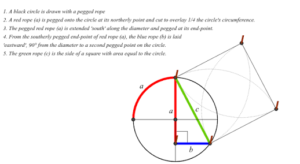# Rajju Ganit and how we are surprised to learn about ancient mathematics

Rajju ganit (string geometry, cord geometry) aims to teach practical geometry more understandably.

The useful new things students would learn as part of string geometry or Rajju Ganit are

• Conceptual clarity.
• Measurement of angles
• Simplified geometry
• Measurement of the circle
• The theory of approximation.
• Trigonometry.
• Applications to real life.

There are two main new features:

(1) The cord replaces the entire compass box.

(2) Empirical methods are admitted in geometry contrary to the philosophy of formal math and using instead the philosophy of approximation.

As a part of the learning session through Rajju Ganit, Children used a rope to draw a circle, measure the circumference of the circle, and find the value of Pi.

The circumference of the circle is equal to the length of its boundary. This means that the perimeter of a circle is equal to its circumference. The length of the rope that wraps around the circle’s boundary perfectly will be equal to its circumference. The below-given figure helps you visualize the same. The circumference can be measured by using the given formula:

Circumference of a circle = 2πR =  π D

where ‘r’ is the radius of the circle and π is the mathematical constant whose value is approximated to 3.14 or 22/7. The circumference of a circle can be used to find the area of that circle.

For a circle with radius ‘r’ and circumference ‘C’:

• π = Circumference/Diameter
• π = C/2r = C/d
• C = 2πrSimilarly using the rope, a circle and a square of the same area can be constructed and observed.

Squaring the circle can be done easily using the Rajju ganit method.